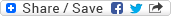## Thursday, May 22, 2008

### Adobe - Larger of two int without Logical or Relational ops

Question: Find the larger of two integers without using logical or relational operators.

Note: This question was supposedly asked sometime back in the 'C Programming' section of the written test for a Dev position at Adobe. The question is purely based on memory and collected either from friends, colleagues, or Web. No guarantee whether the same question actually appeared in their test. However, you may use it to your advantage by having a feel of the type of questions Adobe asks.

Solution: In C, UINT_MAX represents the maximum value an unsigned integer can have and INT_MAX represents the maximum value of an integer variable.

So, (UINT_MAX >> 1) will have the same value as INT_MAX and consequently ~(UINT_MAX >> 1) = ~(INT_MAX) with all the bits as '0' and only the sign bit set as '1'.

If the given two integers are 'a' and 'b' then (a - b) will be negative for the case where 'a' < 'b' and hence the sign bit will be '1' for (a - b) in that case. Right? Now (a - b) & ~(UINT_MAX >> 1) will be 'true (1)' in this case as for both the operands of the operator '&', the leftmost bit is '1'. Other bits will become '0' as the second operand has all other bits set as '0'.

That means (a - b) & ~(UINT_MAX >> 1) will be true in case 'a' < 'b'. We can easily understand that (a - b) will be true (some positive number) in case of 'a' > 'b'. Otherwise, both the operands will be equal i.e., 'a' == 'b' in which case both the above conditions will return 'false (0)'.

Code Snippet in C:

if ((a - b) & ~(UINT_MAX >> 1)) /*... OR, (a - b) & ~INT_MAX ...*/
/*... a is less than b ...*/
else if (a - b)
/*... a is greater than b ...*/
else
/*... a is equal to b ...*/#### 1 comment:

Unknown said...

Tricky use of bit wise and shift ops. Difficult to think particularly in interviews or written tests. Needs practice.